Maths-
General
Easy

Question

# A plate of 3 cm thick, 9 cm broad, and 27 m long is melted into a cube. find the difference in surface area of the two solids correct to the nearest whole number.

## 37293927Hint:

## The correct answer is: 27

General
Maths-

### Which number is next in the sequence?35, 85, 135, 185, __

Hint:- A sequence of numbers which has a common difference between any two consecutive numbers is called an arithmetic progression (A.P.). The arithmetic progression general form is given by a, a + d, a + 2d, a + 3d, . . .. Hence, the formula to find the nth term is:
an = a + (n – 1) × d
Solution :- We have given the pattern of numbers

35, 85, 135, 185, __
We have to find the next number.
We can see clearly that it is an Arithmetic progression With Common difference = 85 - 35 = 50
= 135 - 85 = 50
= 185 - 135 = 50
Therefore next term will be
185 + Common difference
185 + 50 = 235
Therefore, the pattern will be
35, 85, 135, 185, 235

### Which number is next in the sequence?35, 85, 135, 185, __

Maths-General
Hint:- A sequence of numbers which has a common difference between any two consecutive numbers is called an arithmetic progression (A.P.). The arithmetic progression general form is given by a, a + d, a + 2d, a + 3d, . . .. Hence, the formula to find the nth term is:
an = a + (n – 1) × d
Solution :- We have given the pattern of numbers

35, 85, 135, 185, __
We have to find the next number.
We can see clearly that it is an Arithmetic progression With Common difference = 85 - 35 = 50
= 135 - 85 = 50
= 185 - 135 = 50
Therefore next term will be
185 + Common difference
185 + 50 = 235
Therefore, the pattern will be
35, 85, 135, 185, 235

General
General

### A cuboidal water tank is 6m long , 5m wide and 4.5 m deep.How many litres of water can it hold ?

Step-by-step solution:
The given cuboidal water tank has-
• Length = 6m,
• Width = 5m and
• Height = 4.5m
Now, we know that-
Volume of a cuboid = Length * Width * Height
∴ Volume of the cuboidal tank = 6 * 5 * 4.5
∴ Volume of the cuboidal tank = 135 m3.
Now, we know that-
1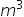= 1,000 L
∴ 135= 1,000 * 135
∴ 135= 1,35,000 L.

### A cuboidal water tank is 6m long , 5m wide and 4.5 m deep.How many litres of water can it hold ?

GeneralGeneral
Step-by-step solution:
The given cuboidal water tank has-
• Length = 6m,
• Width = 5m and
• Height = 4.5m
Now, we know that-
Volume of a cuboid = Length * Width * Height
∴ Volume of the cuboidal tank = 6 * 5 * 4.5
∴ Volume of the cuboidal tank = 135 m3.
Now, we know that-
1= 1,000 L
∴ 135= 1,000 * 135
∴ 135= 1,35,000 L.
General
Maths-

### Show the conjecture is false by finding a counterexample.Two supplementary angles form a linear pair.

Solution:-
Given, two supplementary angles always form a liner pair

We have to determine if the given statement is true or false.
When the sum of measures of two angles is 180 degrees, then the angles are called supplementary angles.
When two lines intersect each other at a single point, linear pairs of angles are formed.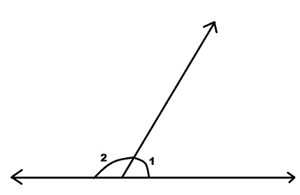From the figure,
∠1 + ∠2 = 180°
Linear pair of angles occurs in a straight line.
So, two supplementary angles do not always form a linear pair.
Therefore, the given statement is false.

### Show the conjecture is false by finding a counterexample.Two supplementary angles form a linear pair.

Maths-General
Solution:-
Given, two supplementary angles always form a liner pair

We have to determine if the given statement is true or false.
When the sum of measures of two angles is 180 degrees, then the angles are called supplementary angles.
When two lines intersect each other at a single point, linear pairs of angles are formed.From the figure,
∠1 + ∠2 = 180°
Linear pair of angles occurs in a straight line.
So, two supplementary angles do not always form a linear pair.
Therefore, the given statement is false.General
Maths-

### Show the conjecture is false by finding a counterexample.Two adjacent angles always form a linear pair

Solution :- We have given the statement
Two adjacent angles always form a linear pair.
We have to prove the statement is false.
The linear pair of angles is a pair of angles which is created by the intersection of two straight lines and the sum of a linear pair of angles is 180°.
Now, if we take an angle which is less than 180° and bisect that angle with the help of a straight line, then we will get two adjacent angles. And, the sum of those two adjacent angles isn't 180°.
In this way, we can come to a conclusion that every pair of adjacent angles will be not considered as the linear pair of angles.
So, the given conjecture is false.

### Show the conjecture is false by finding a counterexample.Two adjacent angles always form a linear pair

Maths-General
Solution :- We have given the statement
Two adjacent angles always form a linear pair.
We have to prove the statement is false.
The linear pair of angles is a pair of angles which is created by the intersection of two straight lines and the sum of a linear pair of angles is 180°.
Now, if we take an angle which is less than 180° and bisect that angle with the help of a straight line, then we will get two adjacent angles. And, the sum of those two adjacent angles isn't 180°.
In this way, we can come to a conclusion that every pair of adjacent angles will be not considered as the linear pair of angles.
So, the given conjecture is false.
General
Maths-

### A cubical tank 50 cm in length and 36 cm in breadth contain water. A cube of x cm edge is dropped into it and fully immersed. If the rise in water level is 15 cm, solve for x. and hence find the T.S.A of the cubical tank

We have given the dimensions of cubical tank
Length l = 50cm
The dimensions of cube dropped are
Side = x cm
We have to find the value of x and TSA of cubical tank.
It is given that rise in water level is 15 cm , so it will be the height of the water in the cubical tank

h = 15 cm
Therefore the volume of the cube dropped will be

Volume = l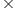h

x3 = 503615

= 27000

As 27000 is a cube of 30

x3 = (30)3

therefore, x = 30
The TSA of the cubical tank = 2[lb + bh + hl]

= 2[(50)(36) + (36)(15) + (15)(50)l]

= 2[1800 + 540 + 750]

= 2

= 6180
Which is approximately equal to 6200.
Therefore, the correct option is a) 30 , 6200.

### A cubical tank 50 cm in length and 36 cm in breadth contain water. A cube of x cm edge is dropped into it and fully immersed. If the rise in water level is 15 cm, solve for x. and hence find the T.S.A of the cubical tank

Maths-General
We have given the dimensions of cubical tank
Length l = 50cm
The dimensions of cube dropped are
Side = x cm
We have to find the value of x and TSA of cubical tank.
It is given that rise in water level is 15 cm , so it will be the height of the water in the cubical tank

h = 15 cm
Therefore the volume of the cube dropped will be

Volume = lh

x3 = 503615

= 27000

As 27000 is a cube of 30

x3 = (30)3

therefore, x = 30
The TSA of the cubical tank = 2[lb + bh + hl]

= 2[(50)(36) + (36)(15) + (15)(50)l]

= 2[1800 + 540 + 750]

= 2

= 6180
Which is approximately equal to 6200.
Therefore, the correct option is a) 30 , 6200.

General
Maths-

### Show the conjecture is false by finding a counterexample.If the product of two numbers is even, then the two numbers must be even.

Solution:-
We have given a statement
If the product of two numbers is even, then the two numbers must be even.
We have to show the given conjecture is false.
Let us take two even numbers 6 and 8
Product of considered numbers = 6 x 8 = 48
Product we get is an even number , so the given statement is true for considered values.
If we consider one odd and one even number , 5 and  6
Product = 5 x 6 = 30
So, the product obtained is an even which contradicts the given statement
So, We can say that if the product of two numbers is even, then the two numbers need be even.
So, the given conjecture is false.

### Show the conjecture is false by finding a counterexample.If the product of two numbers is even, then the two numbers must be even.

Maths-General
Solution:-
We have given a statement
If the product of two numbers is even, then the two numbers must be even.
We have to show the given conjecture is false.
Let us take two even numbers 6 and 8
Product of considered numbers = 6 x 8 = 48
Product we get is an even number , so the given statement is true for considered values.
If we consider one odd and one even number , 5 and  6
Product = 5 x 6 = 30
So, the product obtained is an even which contradicts the given statement
So, We can say that if the product of two numbers is even, then the two numbers need be even.
So, the given conjecture is false.General
Maths-

### Show the conjecture is false by finding a counterexample.If a + b = 0, then a = b = 0.

Solution:-
We have given a statement
If a + b = 0, then a = b = 0
We have to show the given conjecture is false.
Let us take a = 0 and b = 0
Then a + b = 0 + 0 = 0
Given statement is true for considered values of a and b.
Let us take a = 3 and b = - 3
Then a + b = 3 + (- 3) = 3 – 3 = 0
From this example we can say that it is not necessary that and b should be 0 to get sum of a and b to be 0 .
Therefore, the given conjecture is false.

### Show the conjecture is false by finding a counterexample.If a + b = 0, then a = b = 0.

Maths-General
Solution:-
We have given a statement
If a + b = 0, then a = b = 0
We have to show the given conjecture is false.
Let us take a = 0 and b = 0
Then a + b = 0 + 0 = 0
Given statement is true for considered values of a and b.
Let us take a = 3 and b = - 3
Then a + b = 3 + (- 3) = 3 – 3 = 0
From this example we can say that it is not necessary that and b should be 0 to get sum of a and b to be 0 .
Therefore, the given conjecture is false.
General
Maths-

### calculate the surface area of a cube and volume of a cube with the given diagonal length of 15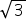cm

We have given the diagonal of the cube
D= 15cm
We have to find the Surface area and volume of the cube.
We know that Diagonal of the cube =a
Where a is side of the cube
Comparing with the given value we get

a = 15 cm
Therefore , Surface area of cube = 6a2

= 6(15)2

= 6(225)

= 1350
And, volume of the given cube = a3

= (15)3

= 3375
Therefore the correct option is c)1350, 3375.

### calculate the surface area of a cube and volume of a cube with the given diagonal length of 15cm

Maths-General
We have given the diagonal of the cube
D= 15cm
We have to find the Surface area and volume of the cube.
We know that Diagonal of the cube =a
Where a is side of the cube
Comparing with the given value we get

a = 15 cm
Therefore , Surface area of cube = 6a2

= 6(15)2

= 6(225)

= 1350
And, volume of the given cube = a3

= (15)3

= 3375
Therefore the correct option is c)1350, 3375.

General
Maths-

### Show the conjecture is false by finding a counterexample.If the product of two numbers is positive, then the two numbers must both be positive.

Solution:-
We have given a statement If the product of two numbers is positive, then the two numbers must both be positive.

If we consider two positive numbers 7 and 9

Then , their product, 7 x 9 = 63

Hence, the statement is correct for positive integers

Suppose we take two negative integers - 7 and - 9

Then, their product, - 7 x - 9 = 63

So,The product of any two positive numbers is positive, and the product of any two negative numbers is also positive.
Therefore, the given statement is false.

### Show the conjecture is false by finding a counterexample.If the product of two numbers is positive, then the two numbers must both be positive.

Maths-General

Solution:-
We have given a statement If the product of two numbers is positive, then the two numbers must both be positive.

If we consider two positive numbers 7 and 9

Then , their product, 7 x 9 = 63

Hence, the statement is correct for positive integers

Suppose we take two negative integers - 7 and - 9

Then, their product, - 7 x - 9 = 63

So,The product of any two positive numbers is positive, and the product of any two negative numbers is also positive.
Therefore, the given statement is false.General
Maths-

### Show the conjecture is false by finding a counterexample.The square root of any positive integer x is always less than x.

Solution: - We have given the statement
The square root of any positive integer x is always less than
Let us take any positive integer x
We consider that the square root of any interger x is always less than x

But if we consider integer 1 then,

The square root of 1 is 1.

Which is not less than 1
So, the given statement is false

### Show the conjecture is false by finding a counterexample.The square root of any positive integer x is always less than x.

Maths-General
Solution: - We have given the statement
The square root of any positive integer x is always less than
Let us take any positive integer x
We consider that the square root of any interger x is always less than x

But if we consider integer 1 then,

The square root of 1 is 1.

Which is not less than 1
So, the given statement is false

General
Maths-

### Show the conjecture is false by finding a counterexample.All prime numbers are odd.

Solution :- We have given a statement that
All prime numbers are odd
Before deciding we will know the definition of prime numbers
Definition - a prime number has only 2 factors - itself and 1.
Hence the smallest natural prime number is 2, and the only on that is even. All other prime numbers are odd, and there are infinitely many prime numbers.
Therefore, it shows that All prime numbers are not odd.

### Show the conjecture is false by finding a counterexample.All prime numbers are odd.

Maths-General
Solution :- We have given a statement that
All prime numbers are odd
Before deciding we will know the definition of prime numbers
Definition - a prime number has only 2 factors - itself and 1.
Hence the smallest natural prime number is 2, and the only on that is even. All other prime numbers are odd, and there are infinitely many prime numbers.
Therefore, it shows that All prime numbers are not odd.
General
Maths-

### Complete the conjecture.The sum of the first n odd positive integers is ____.

Solution :- A sequence of numbers which has a common difference between any two consecutive numbers is called an arithmetic progression (A.P.). The arithmetic progression general form is given by a, a + d, a + 2d, a + 3d, . . .. Hence, the formula to find the nth term is:
an = a + (n – 1) × d
To find the sum of arithmetic progression, we have to know the first term, the number of terms and the common difference between each term. Then use the formula given below:
S = n/2[2a + (n − 1) × d]
To find: Sum of first n odd natural numbers
The first n odd natural numbers are given by 1,3,5,7,9…… (2n – 1) and this forms an AP.
• a = 1
• d = 2
• tn = (2n – 1)
The formula of sum of an A.P series
S = n/2[2a + (n − 1) × d]
S = n /2 [2 + 2n – 2]
S = n /2 [2n]
S = n2
The sum of first n odd natural numbers is n2.

### Complete the conjecture.The sum of the first n odd positive integers is ____.

Maths-General
Solution :- A sequence of numbers which has a common difference between any two consecutive numbers is called an arithmetic progression (A.P.). The arithmetic progression general form is given by a, a + d, a + 2d, a + 3d, . . .. Hence, the formula to find the nth term is:
an = a + (n – 1) × d
To find the sum of arithmetic progression, we have to know the first term, the number of terms and the common difference between each term. Then use the formula given below:
S = n/2[2a + (n − 1) × d]
To find: Sum of first n odd natural numbers
The first n odd natural numbers are given by 1,3,5,7,9…… (2n – 1) and this forms an AP.
• a = 1
• d = 2
• tn = (2n – 1)
The formula of sum of an A.P series
S = n/2[2a + (n − 1) × d]
S = n /2 [2 + 2n – 2]
S = n /2 [2n]
S = n2
The sum of first n odd natural numbers is n2.General
Maths-

### A square is drawn with the length of the side equal to the diagonal of the cube. if the square area is 72075 cm2, then find the side of the cube?

Hint:-
Area of square=side × side=a²
Diagonal of cube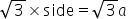unit
Solution:- The length of the square and the diagonal of the cube are coincided. Therefore, the value of the two will be equal.
• We are given that,
Area of the square=72,075 cm²
Side of square=Diagonal of cube
Let the edge of the cube be 'a' cm.
As we know,
length of the diagonal of cube=×edge of the cube=a cm
• The length of the side of the square is equal to the length of the diagonal of the cube.
• Area of square(drawn on diagonal of cube)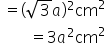• We know that, area of square drawn on the diagonal of a cube= 72,075 cm²
Therefore,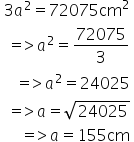Therefore , the side of the cube is 155 cm.

### A square is drawn with the length of the side equal to the diagonal of the cube. if the square area is 72075 cm2, then find the side of the cube?

Maths-General
Hint:-
Area of square=side × side=a²
Diagonal of cubeunit
Solution:- The length of the square and the diagonal of the cube are coincided. Therefore, the value of the two will be equal.
• We are given that,
Area of the square=72,075 cm²
Side of square=Diagonal of cube
Let the edge of the cube be 'a' cm.
As we know,
length of the diagonal of cube=×edge of the cube=a cm
• The length of the side of the square is equal to the length of the diagonal of the cube.
• Area of square(drawn on diagonal of cube)• We know that, area of square drawn on the diagonal of a cube= 72,075 cm²
Therefore,Therefore , the side of the cube is 155 cm.
General
Maths-

### What are all the possible values of b for which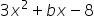is it factorable using only integer coefficients and constant?

Ans:-  {-23,-10,-5,-2,2,5,10,23} are all possible values of b.
Given,is it factorable  so be must be sum of factors of (-8)(3)
Factors of -24 are
(1,-24)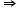b can be -24 + 1 = -23
(2,-12)b can be -12 + 2 = -10
(3,-8)b can be -8 + 3 = -5
(4,-6)b can be 4 - 6 = -2
(6,-4)b can be 6 - 4 = 2
(8,-3)b can be 8 - 3 = 5
(12,-2)b can be 12 - 2 = 10
(24,-1)b can be 24 - 1 = 23
So, there are 8 possible values of b they are {-23, -10, -5, -2, 2, 5, 10, 23}

### What are all the possible values of b for whichis it factorable using only integer coefficients and constant?

Maths-General
Ans:-  {-23,-10,-5,-2,2,5,10,23} are all possible values of b.
Given,is it factorable  so be must be sum of factors of (-8)(3)
Factors of -24 are
(1,-24)b can be -24 + 1 = -23
(2,-12)b can be -12 + 2 = -10
(3,-8)b can be -8 + 3 = -5
(4,-6)b can be 4 - 6 = -2
(6,-4)b can be 6 - 4 = 2
(8,-3)b can be 8 - 3 = 5
(12,-2)b can be 12 - 2 = 10
(24,-1)b can be 24 - 1 = 23
So, there are 8 possible values of b they are {-23, -10, -5, -2, 2, 5, 10, 23}
General
Maths-

### Find a counterexample to show that the following conjecture is false.Conjecture: The square of any integer is always greater than the integer.

We have given, the square of every integer is always greater than the integer.
We have to prove that given statement false.
Number obtained when a number is multiplied by itself three times is called a cube number.
If m = n², then m is a perfect square  where m and n are natural numbers.
Example: consider 1
Square of 1 = 1
Example: consider 2
Square of 2 = 4
Therefore, the square of every natural number is not always greater than the number itself.
Therefore, given statement is false.

### Find a counterexample to show that the following conjecture is false.Conjecture: The square of any integer is always greater than the integer.

Maths-General
We have given, the square of every integer is always greater than the integer.
We have to prove that given statement false.
Number obtained when a number is multiplied by itself three times is called a cube number.
If m = n², then m is a perfect square  where m and n are natural numbers.
Example: consider 1
Square of 1 = 1
Example: consider 2
Square of 2 = 4
Therefore, the square of every natural number is not always greater than the number itself.
Therefore, given statement is false.#### With Turito Foundation.#### Get an Expert Advice From Turito.